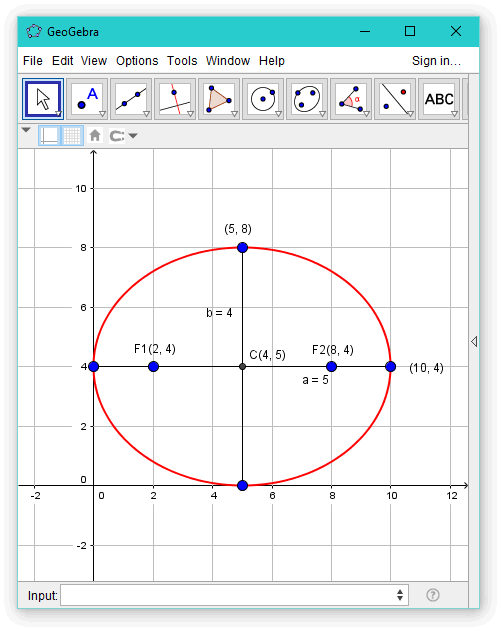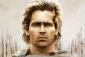# Differential Calculus: center, vertices, foci of the ellipse: 16x² + 25y² - 160x - 200y + 400 = 0

4 posts / 0 new
darbz11
Differential Calculus: center, vertices, foci of the ellipse: 16x² + 25y² - 160x - 200y + 400 = 0

Find the center, vertices, foci of the ellipse given the equation 16x² +25y² -160x - 200y + 400 = 0.

KMST

This is not really calculus. The question might have been answered more promptly, if asked in the algebra section. You also had a fair chance of an answer in the algebra . com website.
16x² +25y² -160x - 200y + 400 = 0
16x² -160x+25y² - 200y = - 400
16(x² -10x)+25(y² - 8y) = - 400
16(x² -10x)+16(25) +25(y² - 8y) +25(16)= - 400+16(25)+25(16)
16(x² -10x+25)+25(y² - 8y+16) = 400
16(x-5)2+25(y-4)2 = 400
16(x-5)2/400+25(y-4)2/400 = 1
(x-5)2/25+(y-4)2/16 = 1
(x-5)2/52+(y-4)2/42 = 1
You can see that the last equation above represents an ellipse
centered at (5,4),
with horizontal major axis,
a semi-major axis a=5 ,
and a semi-minor axis b=4 .
That means that the vertices, at the ends of the major axis, are
(5-5,4) =(0,4) and (5+5,4) = (10,4) .
The co-vertices, at the end of the minor axis, are
(5,4-4) = (5,0) and (5,4+4) = (5,8) .
The foci are on the major axis, between the center and the vertices,
at (5-c,4) and (5+c,4) .
All that is left to do is find the focal distance, c .
For that you may remember that in an ellipse a2 = b2 + c2 ,
or you may remember the definition of ellipse, and deduce the formula yourself.
In this case, substituting the values found for a and b,
52 = 42 + c2 , or
25 = 16 + c2 ---> c2 = 25-16 = 9 ---> c = 3 .
Then, the foci are at
(5-3,4) = (2,4) and (5+3,4) = (8,4) .

Jhun VertUsing Calculus for Vertices
$16x^2 + 25y^2 - 160x - 200y + 400 = 0$

For Upper and Lower Vertices

$32x + 50y\,y' - 160 - 200y' = 0$

At the highest and lowest points, y' = 0
$32x - 160 = 0$

$x = 5$

$16(5^2) + 25y^2 - 160(5) - 200y + 400 = 0$

$25y^2 - 200y = 0$

$y = 8 ~ \text{and} ~ 0$

upper vertex = (5, 8)
lower vertex = (5, 0)

For Left and Right Vertices

$32x\,x' + 50y - 160x' - 200 = 0$

At the extreme left and extreme right points, x' = 0
$50y - 200 = 0$

$y = 4$

$16x^2 + 25(4^2) - 160x - 200(4) + 400 = 0$

$16x^2 - 160x = 0$

$x = 10 ~ \text{and} ~ 0$

right vertex = (10, 4)
left vertex = (0, 4)

Below is the plot to locate the center and other points:AlexanderSir, pwede din bang gamitin ang y' = infinity para kunin ang left and right vertices? In this way kasi, isa na lang ang kukunin, yung y' na lang, hindi na kailangan ang x'.

• Mathematics inside the configured delimiters is rendered by MathJax. The default math delimiters are $$...$$ and $...$ for displayed mathematics, and $...$ and $...$ for in-line mathematics.